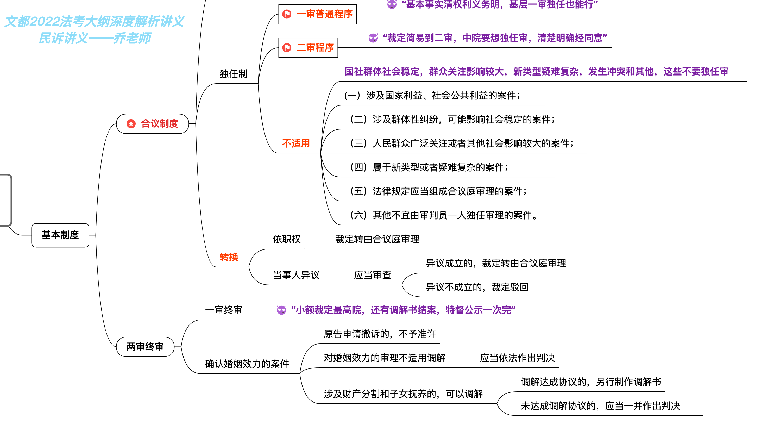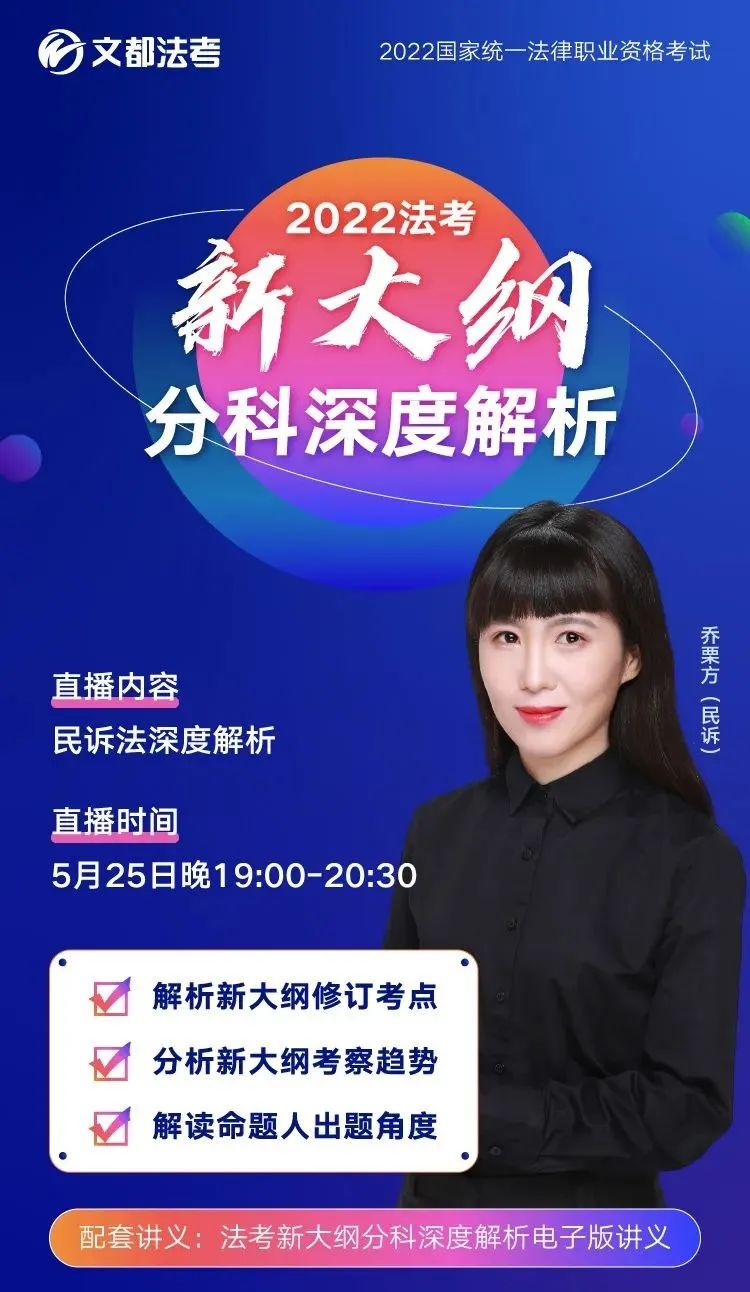<section data-role="paragraph" style="margin: 0px; padding: 0px; outline: 0px; max-width: 100%; box-sizing: border-box !important; word-wrap: break-word !important; font-family: -apple-system, BlinkMacSystemFont, " helvetica="" neue",="" "pingfang="" sc",="" "hiragino="" sans="" gb",="" "microsoft="" yahei="" ui",="" yahei",="" arial,="" sans-serif;="" font-style:="" normal;="" font-variant-ligatures:="" font-variant-caps:="" font-weight:="" letter-spacing:="" 0.544px;="" orphans:="" 2;="" text-indent:="" 0px;="" text-transform:="" none;="" white-space:="" widows:="" word-spacing:="" -webkit-text-stroke-width:="" background-color:="" rgb(255,="" 255,="" 255);="" color:="" rgb(0,="" 0,="" 0);="" font-size:="" medium;="" text-align:="" start;="" visibility:="" visible;"="">

1、解析课程更深入

2、听解析课效率更高

3、前辈说：当年没听我很后悔！

@我看见了风

@这这这儿